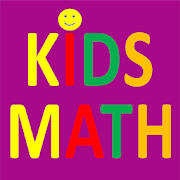# Kids Math: Multiply, Divide, Add, Subtract - Lumos Educational App Store4.41
Price -FREE
\$0

#### DESCRIPTION:

Kids Math, provides free and fun filled Cool Mental Math games for Elementary kids for practicing Addition, Subtraction, Multiplication, and Division. It helps you to increase your Math calculation speed and accuracy. This game is most suitable for kids from age 7 to 12 years. Also, adults who love math will enjoy this game. It may be challenging for grown ups too. There are total 20 free educational games for kids to play and practice addition, subtraction, multiplication, and division ( +,-,/,*). There are total 12 levels for each game. Each level has 10 questions. First four levels are u

#### OVERVIEW:

Kids Math: Multiply, Divide, Add, Subtract is a free educational mobile app By AA learning.It helps students in grades 2 practice the following standards 2.OA.A.1,2.OA.B.2,2.OA.C.3.

This page not only allows students and teachers download Kids Math: Multiply, Divide, Add, Subtract but also find engaging Sample Questions, Videos, Pins, Worksheets, Books related to the following topics.

1. 2.OA.A.1 : Use addition and subtraction within 100 to solve one- and two-step word problems involving situations of adding to, taking from, putting together, taking apart, and comparing, with unknowns in all positions, e.g., by using drawings and equations with a symbol for the unknown number to represent the problem.

2. 2.OA.B.2 : Fluently add and subtract within 20 using mental strategies.

3. 2.OA.C.3 : Determine whether a group of objects (up to 20) has an odd or even number of members, e.g., by pairing objects or counting them by 2s; write an equation to express an even number as a sum of two equal addends.

2

2.OA.A.1
2.OA.B.2
2.OA.C.3

#### ADDITIONAL INFORMATION:

Developer: AA learning

Software Version: 2.9.7.19

Category: Education

### RELATED APPSEdSearch WebSearch